# Help required regarding rule engine

Hi,

I need help regarding the rule engine, is it possible to have output a combination of Col-Value and String (or another Col-Value)

For example, following given an error,

TRUE => \$Col 1\$ + \$Col 2\$
TRUE => \$Col 1\$ + “ABC”

Thanks.

1 Like

The -Rule Engine- node cannot do operations on the result after the “=》” sign.

You would need instead to use the -Column Expressions- node:

Hope it helps

Best
Ael

2 Likes

Thanks for the response,

I have added this node, actually my requirement is something like this,

\$Col 1\$ > \$Col 2\$ AND \$Col 1\$ < \$Col 3\$ => \$Col 1\$ + \$Col 2\$

I am unable to write this conditional statement in Column-Expressions node.

Sorry I’m answering from my mobile phone and cannot provide you with a workflow.

If your columns and operations are all numeric, then you can use the -Math Formula- node which has an “if( condition, then, else)” function.

Hope it helps.

Best
Ael

1 Like

Thanks for the response, I tried it but there is a problem that the output has to be a number not a string.

My requirement is as follows, the output has to a combination of strings (not necessarily as number). [Sorry I did not mention it clearly]

\$Col 1\$ > \$Col 2\$ AND \$Col 1\$ < \$Col 3\$ => \$Col 4\$ + \$Col 5\$ + “Some String”

\$Col 4\$ and \$Col 5\$ are strings.

Please have a look at the following thread which looks very similar to your problem. You will find your solution from @bruno29a’s last post based on the -Column Expression- node.

Best

Ael

1 Like

The Column Expression should be used in this case, as suggested by @aworker .

Rule Engine does not allow operations, only conditions. You can apply conditions, but your results should be either values from an existing column or variable, or hardcoded values, basically “pre-compiled” values.

Also, you can’t have 2 TRUE statements. Well, I don’t know if Knime allows you to, but it’s irrelevant, as even if it did, only the first TRUE statement will be true - the way Rule Engine works is it evaluates your statements in the order you have written them, and as soon as a line is true, then that’s the chosen rule and it stops evaluating the rest of the lines.

With Math Formula (and with String Manipulation), it’s the opposite. It allows you to do operations (and manipulation), but you can’t apply conditions (though there are a few tricks where you can indirectly apply some limited conditions).

The Column Expression (and the Variable Expression) allows you to do both: Apply condition and do operations/manipulation.

1 Like

With the -Math Formula- node you can cause it has an “if(,)” functionConversely and amazingly, the -String Manipulator- node has mathematical functions that the -Math Formula- node hasn’t lol

Best
Ael

Hi @aworker , yes I am aware, but it’s quite limited, and would not work in this case. And I did not want to complicate the explanationEDIT:
Here’s the equivalent of the 2 functions in Math Formula:
toBoolean(x):
`if(x, true, false)`

toDouble(x):
`x * 1.0`

1 Like

Indeed, my comment was just to highlight that it is funny the -String Manipulation- node has “direct” mathematical functions that the Math node does not have, indeed you can reach the same result as you showEdit: How do you implement in the -Math Formula- node the “toLong()” -String Manipulation- node function?

1 Like

Hi @aworker , you can’t convert to Long in Math Formula. You’ve opened a can of worm here… cause it looks like this could be a problem in Math Formula.

Input: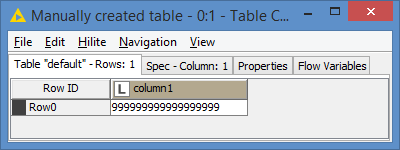Simple Math Formula `\$column1\$ - 1` which should return a Long number:

Results: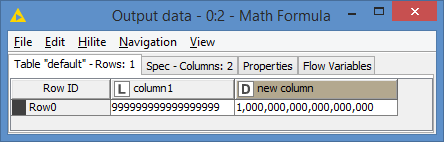1. Test 2:Results: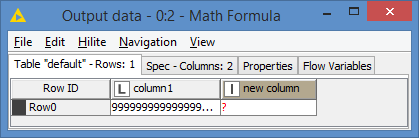1. Test 3: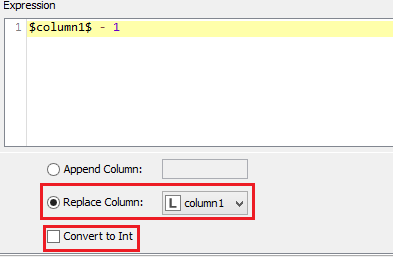Results:1. Test 4: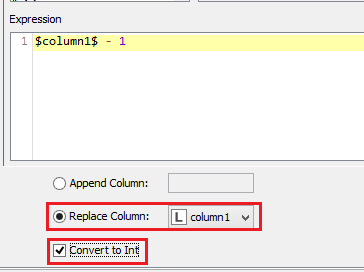Results: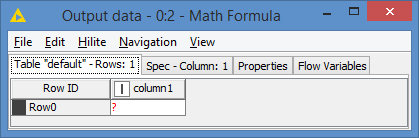You would not even be able to compute this via String Manipulation even if it supports toLong() as you cannot do Math computation in String Manipulation.

The workaround would be to use Column Expressions.

And another thing, why am I getting 1000000000000000000 as result? Shouldn’t it be 999999999999999998 ?

1 Like

Amazingly one can do a bit of math calculation in the -String Manipulation- node.
For instance it is possible to do + - * / simple operators and even < > = boolean operators too.

For instance, the following mathematical formula works and returns “false” as a string:

This string node is really amazing, I love itThe + acts as an addition? I would have thought it would act more as a concatenation (join()) given they’re strings. And you can perform the other operations too? Wow!

Nice find @aworker , I guess you could do deal with Long numbers via the String Manipulation then

1 Like

Thanks for the reponse,

I will explore it. Thank you for your time.

1 Like

Thank you for your efforts as well.1 Like

This topic was automatically closed 7 days after the last reply. New replies are no longer allowed.